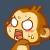﻿ while ((double)clock() / CLOCKS_PER_SEC – start | Code Bye

# while ((double)clock() / CLOCKS_PER_SEC – start

5年前 (2016-04-26) 459次浏览
inline void hello()
{
cout<<“\n\n\n\t\t\t贪吃蛇游戏即将开始!”;                        //准备开始
double start;
for (int i = 3; i >= 0; i–)
{
start = (double)clock() / CLOCKS_PER_SEC;                //得到程序目前为止运行的时间
while ((double)clock() / CLOCKS_PER_SEC – start <= 1);    //经过1秒之后
if (i > 0)
{
system(“cls”);                                        //清屏
cout<<“\n\n\n\t\t\t进入倒计时：”<<i<<endl;                //倒计时
}
else
Update(map, grade, length, autotime);                //刷新地图
}
}

40

20

start = (double)clock() / CLOCKS_PER_SEC;                //得到程序目前为止运行的时间
//此时clock()函数wrap around了
while ((double)clock() / CLOCKS_PER_SEC – start <= 1);    //经过XXXXXX秒之后……CodeBye 版权所有丨如未注明 , 均为原创丨本网站采用BY-NC-SA协议进行授权 , 转载请注明while ((double)clock() / CLOCKS_PER_SEC – start

[1034331897@qq.com]